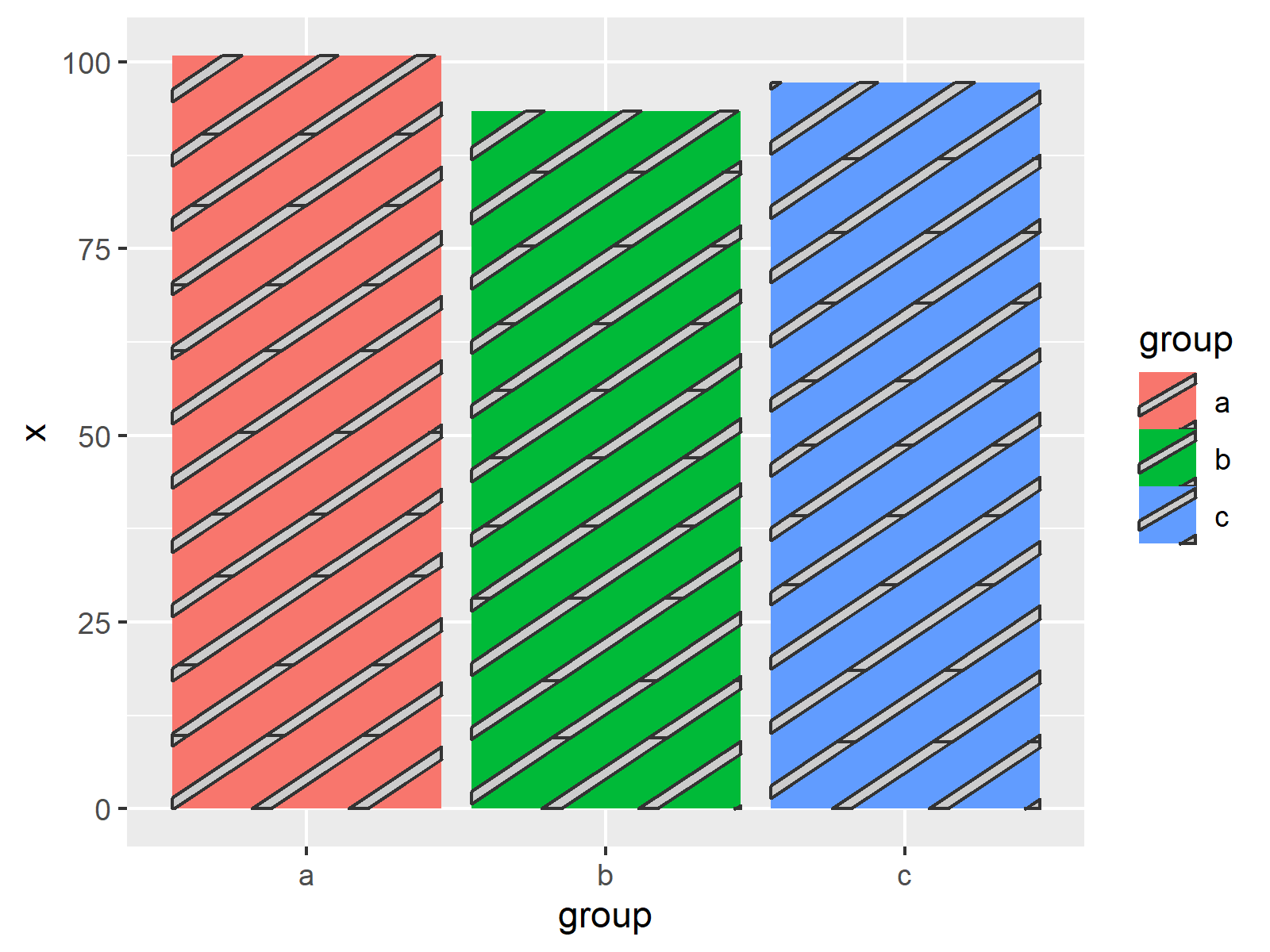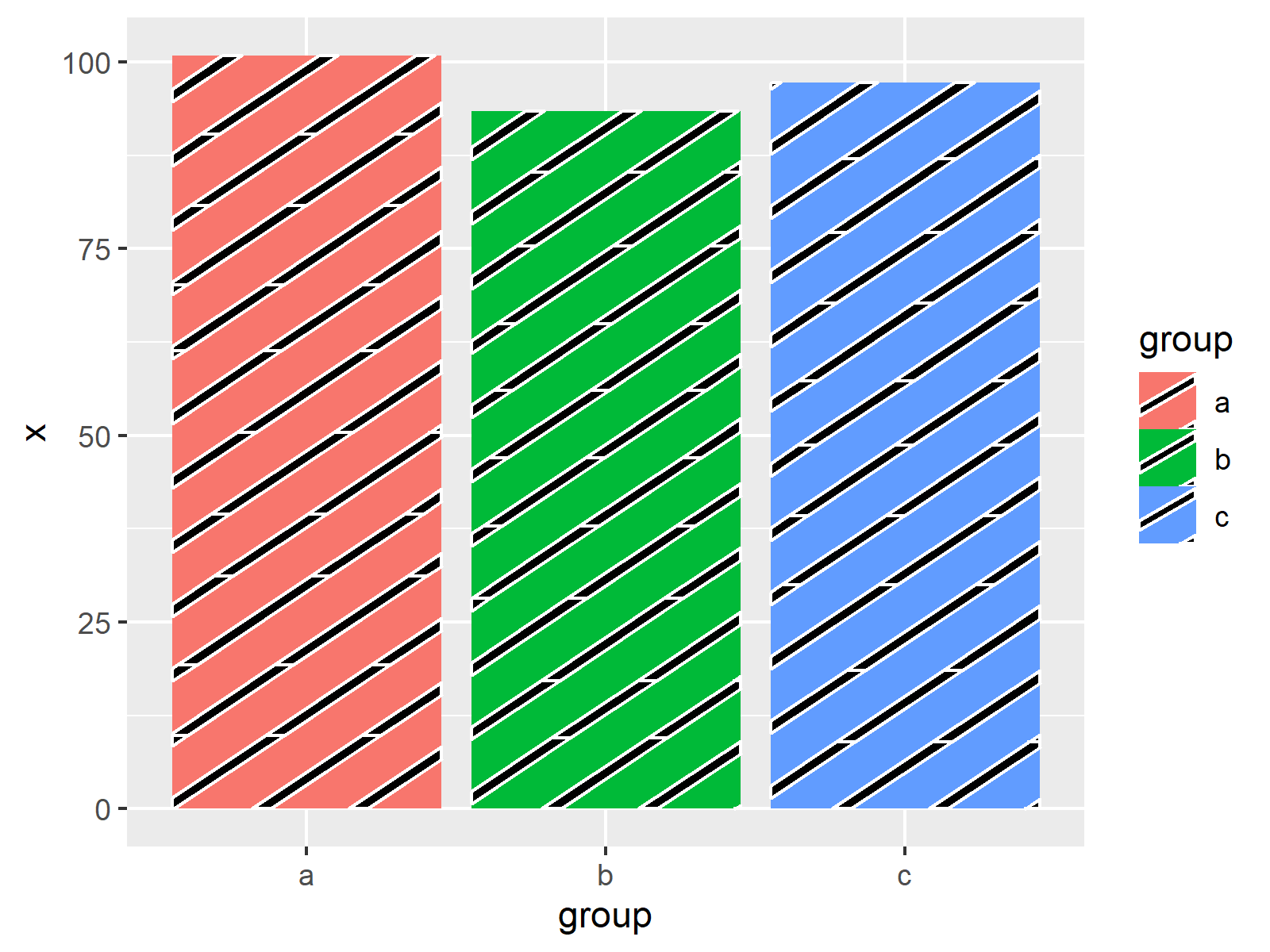# Introduction to ggpattern Package in R (6 Examples) | ggplot2 Plots with Textures

This tutorial explains how to draw ggplot2 plots with textures and patterns using the ggpattern package in R programming.

Let’s dig in…

## Some Details About the ggpattern Package

The ggpattern package is used to add geometric and image-based patterns to ggplot2 plots in R.

If you have never heard about the ggpattern package, you may ask: Why do I even need this package?

The reason: This package uplifts data visualizations with the ggplot2 package to the next level!

Especially in case you want to draw different data groups in the same graphic, it might be helpful to have another way (in addition to colors) to differentiate between the different groups in your data.

Sounds good? So how can we install the ggpattern package?

Unfortunately, the ggpattern package is not on CRAN yet (at least the point when I was writing this tutorial). For that reason we have to install the ggpattern package via GitHub:

```install.packages("remotes") # Install remotes package remotes::install_github("coolbutuseless/ggpattern") library("ggpattern") # Load ggpattern package```

If we want to plot our data with the ggplot2 package, we also have to install and load ggplot2:

```install.packages("ggplot2") # Install ggplot2 package library("ggplot2") # Load ggplot2 package```

Before we jump into the examples of this tutorial, I also want to give credit to Mike FC, the developer of this package. On this homepage, you can find the development process and many additional tutorials on the ggpattern package.

## Example Data

The following data will be used as basement for this R tutorial:

```set.seed(92634) # Create example data data <- data.frame(x = rnorm(30, 10), group = letters[1:3]) head(data) # Head of example data # x group # 1 9.795025 a # 2 9.442812 b # 3 8.954915 c # 4 9.456803 a # 5 7.709386 b # 6 9.589968 c```

Have a look at the previous output of the RStudio console. It reveals that our example data has two columns.

The variable x contains randomly distributed numeric values and the variable group defines three different groups in our data.

Let’s draw these data!

## Example 1: Drawing Barplot with Pattern Using geom_bar_pattern Function

In Example 1, I’ll show how to create a ggplot2 barchart with the default pattern used by the ggpattern package.

For this, we simply have to replace the geom_bar function of the ggplot2 package by the geom_bar_pattern function of the ggpattern package:

```ggplot(data, aes(group, x, fill = group)) + # Create barplot with pattern geom_bar_pattern(stat = "identity")```Figure 1 illustrates the output of the previous R programming syntax: A ggplot2 barplot with the same texture in each bar.

## Example 2: Manually Specify Colors of Pattern Using pattern_color & pattern_fill Arguments

In Example 2, I’ll illustrate how to change the colors of our pattern.

We can use the pattern_color argument to specify the borders and the pattern_fill argument to edit the filling color of our pattern.

```ggplot(data, aes(group, x, fill = group)) + # Modify colors of pattern geom_bar_pattern(stat = "identity", pattern_color = "white", pattern_fill = "black")```As shown in Figure 2, the previous syntax created another barchart with patterns, but this time the colors of the patterns were set manually.

## Example 3: Drawing Barplot with Pattern for Each Group Using aes Function & pattern Argument

In my opinion, this example shows the most important point of the ggpattern package!

The syntax below shows how to specify a different texture for each of the groups in our data frame. For this, we have to use the aes function and the pattern argument as shown below:

```ggplot(data, aes(group, x, fill = group)) + # Different pattern for each group geom_bar_pattern(stat = "identity", pattern_color = "white", pattern_fill = "black", aes(pattern = group))```As shown in Figure 3, the previous code drew a barplot with different pattern in each bar. Looks great, doesn’t it?!

## Example 4: Drawing Density Plot with Pattern Using geom_density_pattern Function

So far, we have used the ggpattern package only for barcharts.

This example explains how to draw multiple ggplot2 densities in the same graphic with different patterns for each density using the geom_density_pattern function.

```ggplot(data, aes(x, fill = group)) + # Create density plot with pattern geom_density_pattern(pattern_color = "white", pattern_fill = "black", aes(pattern = group))```As shown in Figure 4, the previous R programming syntax created a graph showing multiple densities with different textures.

## Example 5: Drawing Transparent Density Plot with Pattern Using alpha & pattern_alpha Arguments

In graphics containing multiple overlapping densities, it might be useful to make the densities transparent.

For this, we can use the alpha argument provided by the ggplot2 package in combination with the pattern_alpha argument provided by the ggpattern package.

Have a look at the following R code and its output:

```ggplot(data, aes(x, fill = group)) + # Transparent density plot with pattern geom_density_pattern(pattern_color = "white", pattern_fill = "black", alpha = 0.5, pattern_alpha = 0.5, aes(pattern = group))```Figure 5 shows the previously created transparent density plot.

## Example 6: Drawing Boxplot with Pattern Using geom_boxplot_pattern Function

The following syntax explains how to apply the geom_boxplot_pattern function to draw multiple ggplot2 boxplots with patterns in the same graph:

```ggplot(data, aes(x, fill = group)) + # Create boxplot with pattern geom_boxplot_pattern(pattern_color = "white", pattern_fill = "black", aes(pattern = group))```The output of the previous code is shown in Figure 6 – A graphic containing several boxplots. Each boxplot is shown with a different pattern.

## Video, Further Resources & Summary

Do you need more explanations on the R programming codes of this page? Then you might want to watch the following video of my YouTube channel. I explain the R programming codes of this tutorial in the video tutorial:

Please accept YouTube cookies to play this video. By accepting you will be accessing content from YouTube, a service provided by an external third party.If you accept this notice, your choice will be saved and the page will refresh.

Besides that, you may want to read some of the related articles on my homepage:

Summary: You learned in this tutorial how to apply the functions of the ggpattern package in R. Don’t hesitate to tell me about it in the comments section, in case you have further questions.

Subscribe to the Statistics Globe Newsletter

•Alexis
December 4, 2020 1:29 pm

Hi Joachin, thanks for your script and notes. I’m Alexis.
I was looking forward to install ggpattern and test with ggplot2 but after few hours it was no possible and this is message I got:
> remotes::install_github(“coolbutuseless/ggpattern”)

Any suggestion.
Thanks a best regards!
Alexis

• Hey Alexis,

Thank you for your message. Could you check if you have installed the newest version of R?

Regards,

Joachim

•Erik
August 30, 2021 5:25 am

The official ggpattern repository advises you to make sure you install a bunch of dependencies prior to install ggpattern itself. This way, you will know exactly where your installation is getting stuck. In my case, I had to install some system-wide libraries first, so I could install R dependencies, so I could finally get ggpatterns running.

“`
install.packages(‘ggplot2’)
install.packages(‘scales’)
install.packages(‘grid’)
install.packages(‘glue’)
install.packages(‘rlang’)
install.packages(‘sf’)
install.packages(‘png’)

# install.packages(“remotes”)
remotes::install_github(“trevorld/gridpattern”)
remotes::install_github(“coolbutuseless/ggpattern”)
“`

https://github.com/coolbutuseless/ggpattern/

• Thanks for jumping in Erik, I’m certain this will help many readers!

•SHANTANU TAMULY
August 25, 2021 11:53 am

The number of patterns it can generate is not more than 3. So, if you have more than 3 groups, there use to be the repetition of patterns. Any suggestions?
Regards,
Shantanu.

•William Jacob Pitt
September 10, 2021 2:15 am

Hello!

Thank you very much for the helpful information. I have used ggpattern for a manuscript I’m working on. Do you know how to properly cite ggpattern?

• Hey William,

Thank you for the kind feedback, glad you found the tutorial helpful!

I guess I would cite this link as online resource: https://coolbutuseless.github.io/package/ggpattern/ I’m afraid there is no perfect solution in this specific case, since the package is not on CRAN yet.

Regards

Joachim

•William Jacob Pitt
September 10, 2021 2:50 pm

Sounds great. Thank you!

• Glad to hear that! 🙂

•Valentina Vellani
September 23, 2021 2:46 pm

Hi, is there a way to specify for each boxplot the patterns and color?
I would need to have, let’s say, “circle” in the first box, “stripes” in the second and so on..

Thank you!!